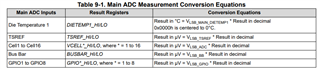If you have a related question, please click the "Ask a related question" button in the top right corner. The newly created question will be automatically linked to this question.

# BQ79616EVM-021: How to calculate GPIO and Dietemp values ?

Part Number: BQ79616EVM-021
Other Parts Discussed in Thread: BQ79616

I am using BQ79616 EVM with MCU, and i am reading Cell voltages along with Dietemp, tsref and GPIOs. i am getting cell voltages pretty good. but the TSREF value is 29450, i have Enabled TSREF from CONTROL2.

For GPIOs i am getting all GPIOs values as 32768, and for Ditemp1 it is 1323 and Dietemp2 is 32768.

How do i calculate dietemp value to represent in degree celsius and same for GPIOs?

ALso how do i represent TSREF ?

• Hello,

The table below from the BQ79616 datasheet should give steps for all calculations you requested above.In case this table is still confusing, I have elaborated on each step for GPIO calculation below.  To calculate voltage from the raw GPIO#_HI/LO registers, use the following calculation steps:

• Convert total value (HI and LO registers) from hex to decimal.
• Ex: if HI has value 3E, and LO has value EF, then the total hex is 3EEF, or decimal 16,111
• Covert LSB (LO register value) from hex to decimal.
• Ex: if LO register has value EF, then the decimal value would be 239
• Multiply HI decimal value and LO decimal value together.
• Ex: using the 3E and EF values from before, multiply 16,111 * 239 = 3850529
• The resulting value is in uV, which is not very convenient.  Divide the number by 1000000 to convert to V.
• Ex: 3850529/1000000 = 3.85V

For TSREF, if you are using the correct steps and getting odd values for TSREF, please directly measure the TSREF voltage using a multimeter to ensure the voltage you are anticipating is present.

Best,

Andria